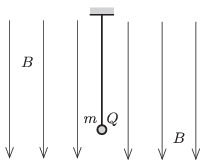Mathematical and Physical Journal
for High Schools
Issued by the MATFUND Foundation
 Already signed up? New to KöMaL?

#Problem P. 4713. (February 2015)

P. 4713. A small ball of mass $\displaystyle m$ and of charge $\displaystyle Q$ is hanging on a piece of thread in uniform vertical magnetic field of magnetic induction $\displaystyle B$. The ball is given an initial velocity such that it undergoes uniform circular motion in a horizontal plane. At another occasion the ball is started such that it moves in the magnetic field along the same radius of circle in the horizontal, but in the opposite direction as it did in the previous case.$\displaystyle a)$ Determine the ratio of the two tensions in the thread in the two cases.

$\displaystyle b)$ What is the difference between the magnitudes of the angular speeds of the two motions?

(4 pont)

Deadline expired on March 10, 2015.

### Statistics:

 27 students sent a solution. 4 points: Asztalos Bogdán, Balogh Menyhért, Bartók Imre, Berta Dénes, Blum Balázs, Bugár 123 Dávid, Csire Roland, Csorba Benjámin, Fehér Balázs, Fekete Panna, Forrai Botond, Fülöp Erik, Iván Balázs, Kasza Bence, Körmöczi Dávid, Körtefái Dóra, Mándoki László, Marosvári Kristóf, Németh Flóra Boróka, Olosz Balázs, Radnai Bálint, Sal Kristóf, Varga-Umbrich Eszter, Varju Ákos, Wiandt Péter. 3 points: Kaposvári Péter. 1 point: 1 student.

Problems in Physics of KöMaL, February 2015